# Chapter 7 Stoichiometry Calculations with Chemical Equations Stoichiometry

• Slides: 36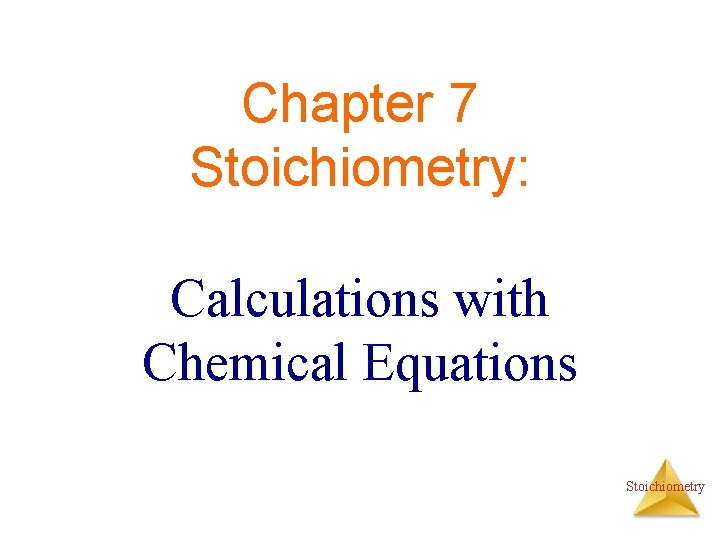Chapter 7 Stoichiometry: Calculations with Chemical Equations StoichiometryStoichiometric Calculations The coefficients in the balanced equation give the ratio of moles of reactants and products StoichiometryUsing coefficients to deermine mole ratio 4 Fe + 3 O 2 2 Fe 2 O 3 How many moles of Fe 2 O 3 are produced when 6. 0 moles O 2 react? 6. 0 mol O 2 x mol Fe 2 O 3 = 4. 0 mol Fe 2 O 3 mol O 2 Stoichiometry 3Learning Check 2 4 Fe + 3 O 2 2 Fe 2 O 3 How many moles of Fe are needed to react with 12. 0 mol of O 2? 1) 3. 00 mol Fe 2) 9. 00 mol Fe 3) 16. 0 mol Fe Stoichiometry 4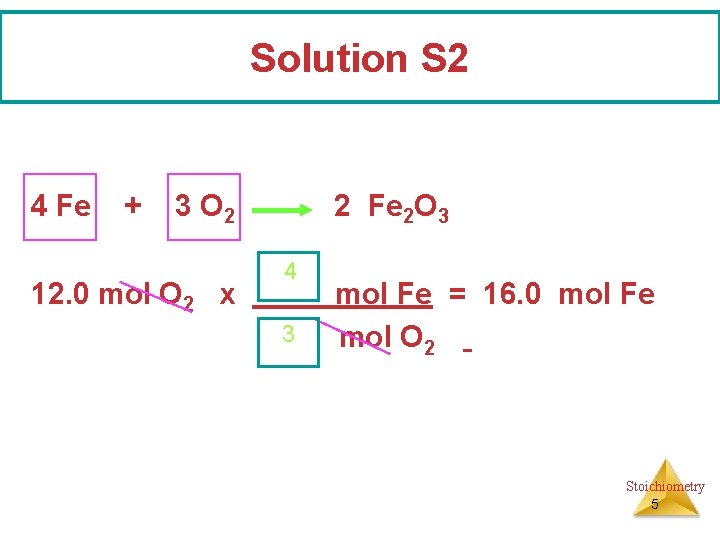Solution S 2 4 Fe + 3 O 2 12. 0 mol O 2 x 2 Fe 2 O 3 4 3 mol Fe = 16. 0 mol Fe mol O 2 Stoichiometry 5Converting mass and moles 4 Fe + 3 O 2 2 Fe 2 O 3 How many grams of O 2 are needed to produce 0. 400 mol of Fe 2 O 3? 1) 38. 4 g O 2 2) 19. 2 g O 2 3) 1. 90 g O 2 Stoichiometry 6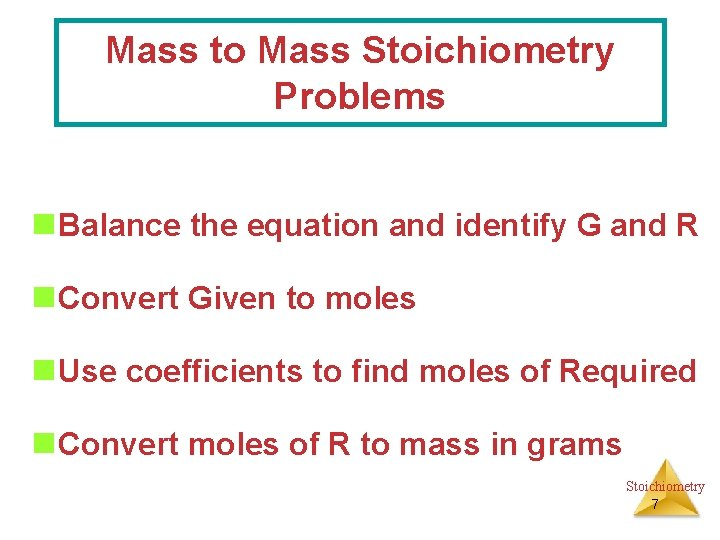Mass to Mass Stoichiometry Problems n Balance the equation and identify G and R n Convert Given to moles n Use coefficients to find moles of Required n Convert moles of R to mass in grams Stoichiometry 7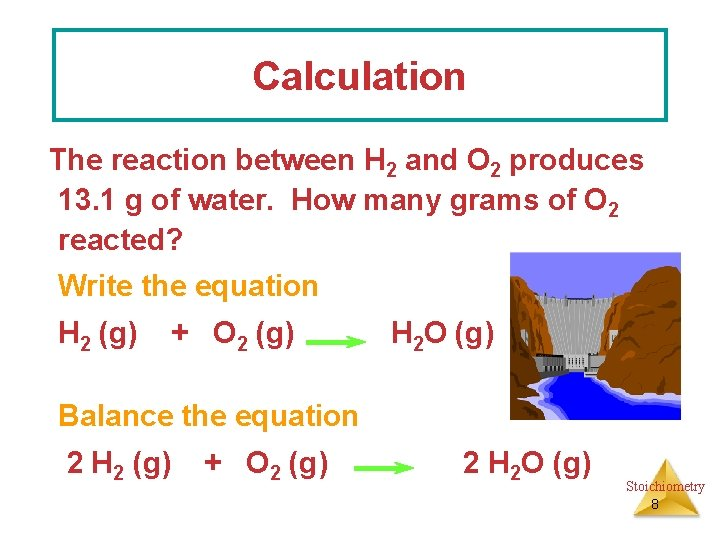Calculation The reaction between H 2 and O 2 produces 13. 1 g of water. How many grams of O 2 reacted? Write the equation H 2 (g) + O 2 (g) H 2 O (g) Balance the equation 2 H 2 (g) + O 2 (g) 2 H 2 O (g) Stoichiometry 8Organize data mol bridge 2 H 2 (g) Plan + O 2 (g) ? g g H 2 O mol H 2 O 2 H 2 O (g) 13. 1 g mol O 2 Setup 13. 1 g H 2 O x 1 mol O 2 x 32. 0 g O 2 8. 0 g H 2 O 2 mol H 2 O 1 mol O 2 = 11. 6 g O 2 Stoichiometry 9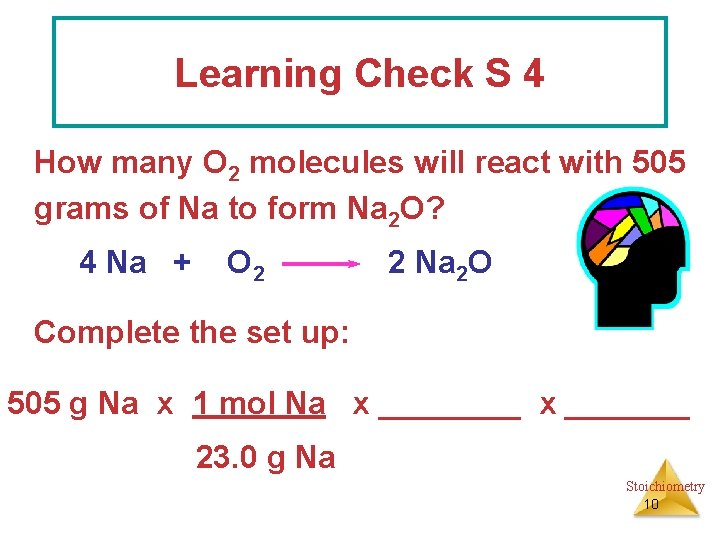Learning Check S 4 How many O 2 molecules will react with 505 grams of Na to form Na 2 O? 4 Na + O 2 2 Na 2 O Complete the set up: 505 g Na x 1 mol Na x _______ 23. 0 g Na Stoichiometry 10Solution S 4 4 Na + O 2 2 Na 2 O 505 g Na x 1 mol O 2 x 6. 02 x 1023 23. 0 g Na 4 mol Na 1 mol O 2 = 3. 30 x 1024 molelcules Stoichiometry 11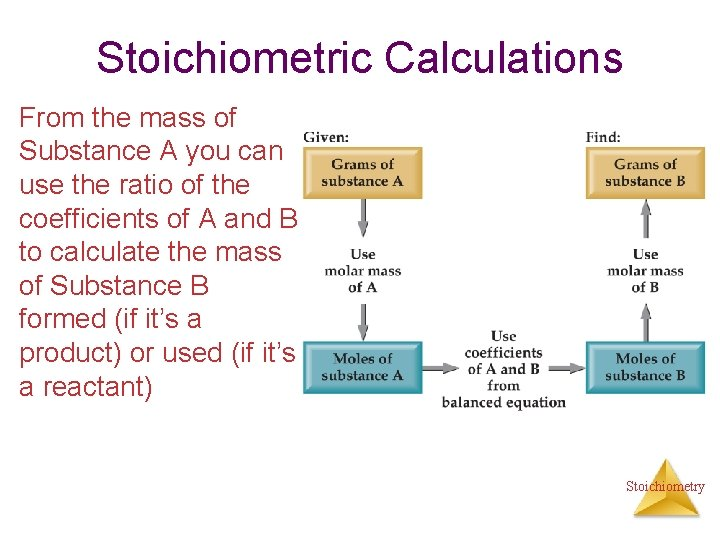Stoichiometric Calculations From the mass of Substance A you can use the ratio of the coefficients of A and B to calculate the mass of Substance B formed (if it’s a product) or used (if it’s a reactant) Stoichiometry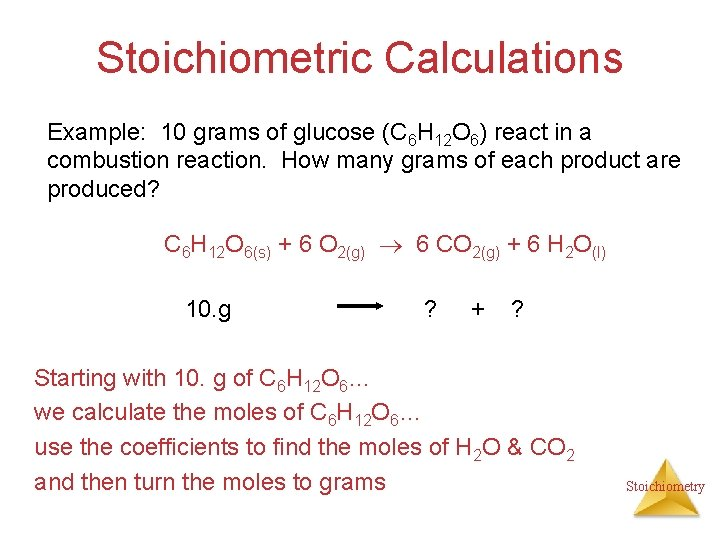Stoichiometric Calculations Example: 10 grams of glucose (C 6 H 12 O 6) react in a combustion reaction. How many grams of each product are produced? C 6 H 12 O 6(s) + 6 O 2(g) 6 CO 2(g) + 6 H 2 O(l) 10. g ? + ? Starting with 10. g of C 6 H 12 O 6… we calculate the moles of C 6 H 12 O 6… use the coefficients to find the moles of H 2 O & CO 2 and then turn the moles to grams StoichiometryStoichiometric calculations C 6 H 12 O 6 + 10. g MW: 180 g/mol 6 O 2 6 CO 2 ? + + 44 g/mol 6 H 2 O ? 18 g/mol #mol: 10. g(1 mol/180 g) 0. 055 mol #grams: 6(. 055) 6(. 055 mol)44 g/mol 6(. 055 mol)18 g/mol 15 g 5. 9 g Stoichiometry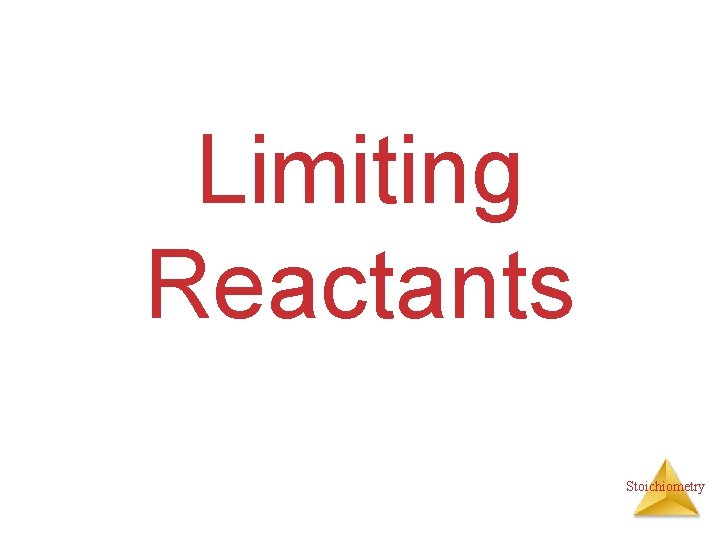Limiting Reactants Stoichiometry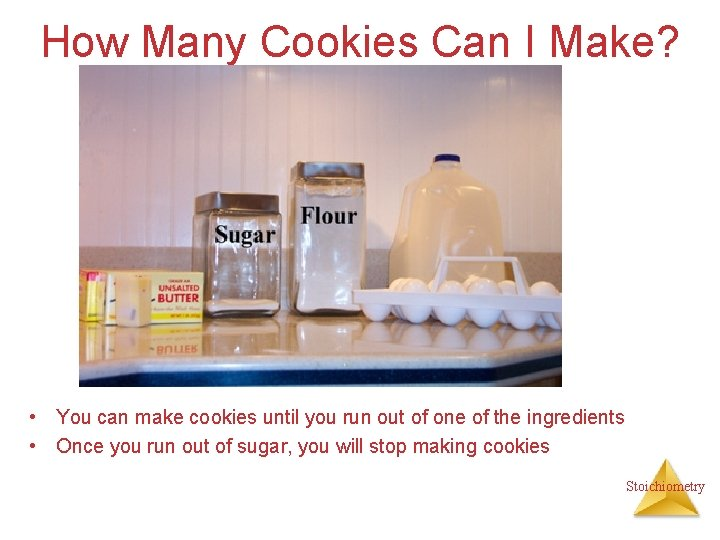How Many Cookies Can I Make? • You can make cookies until you run out of one of the ingredients • Once you run out of sugar, you will stop making cookies Stoichiometry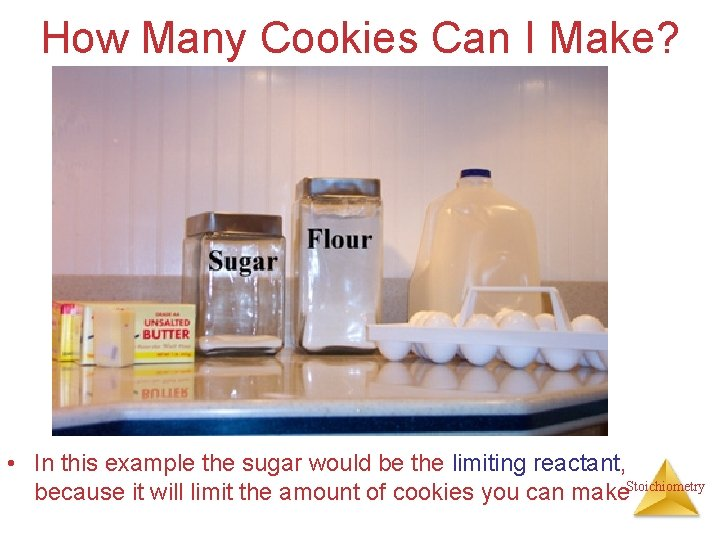How Many Cookies Can I Make? • In this example the sugar would be the limiting reactant, because it will limit the amount of cookies you can make. Stoichiometry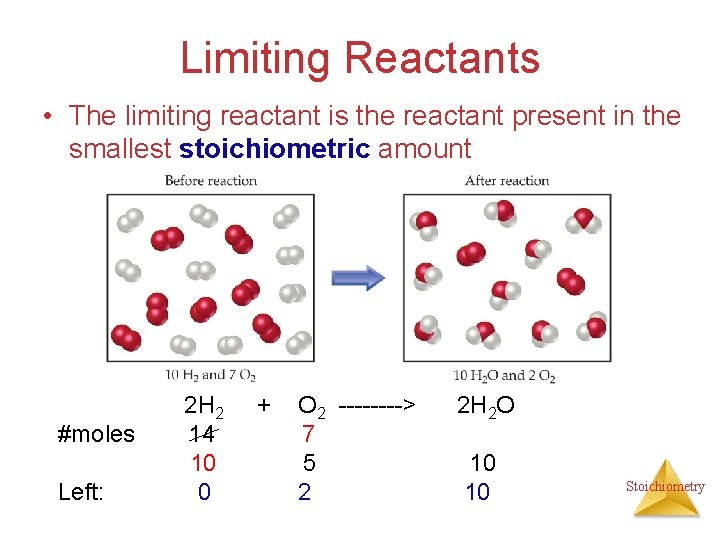Limiting Reactants • The limiting reactant is the reactant present in the smallest stoichiometric amount #moles Left: 2 H 2 14 10 0 + O 2 ----> 7 5 2 2 H 2 O 10 10 StoichiometryLimiting Reactants In the example below, the O 2 would be the excess reagent Stoichiometry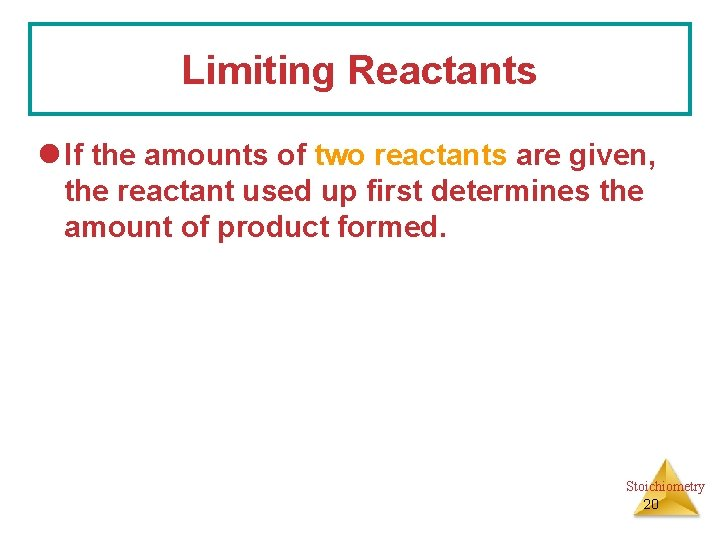Limiting Reactants l If the amounts of two reactants are given, the reactant used up first determines the amount of product formed. Stoichiometry 20Analogy Suppose you are preparing cheese sandwiches. Each sandwich requires 2 pieces of bread and 1 slice of cheese. If you have 4 slices of cheese and 10 pieces of bread, how many cheese sandwiches can you make? Stoichiometry 21Cheese Sandwich Products Sandwich 1 + + = Sandwich 2 + + = Stoichiometry 22Learning Check S 6 How many sandwiches can you make? ____ slices of bread + ____ slices of cheese = ____ sandwiches What is left over? ________ What is the limiting reactant? Stoichiometry 23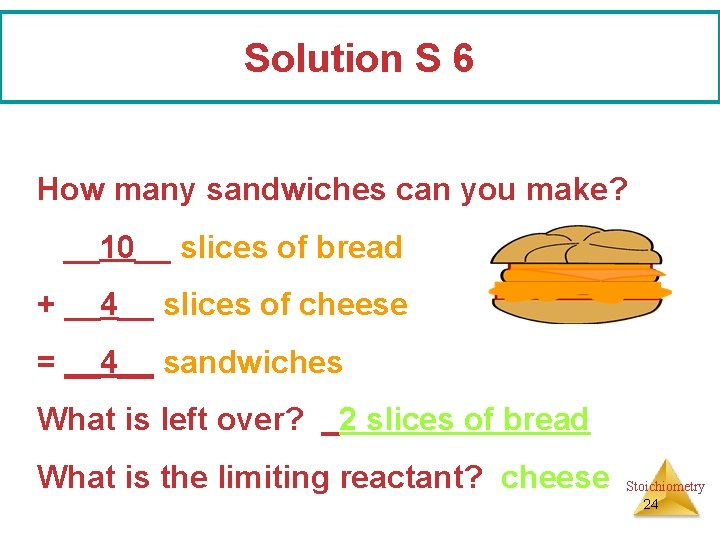Solution S 6 How many sandwiches can you make? __10__ slices of bread + __4__ slices of cheese = __4__ sandwiches What is left over? _2 slices of bread What is the limiting reactant? cheese Stoichiometry 24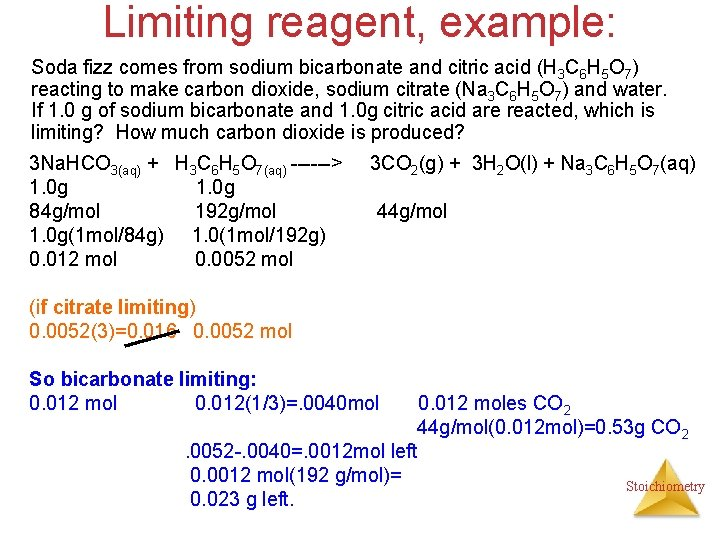Limiting reagent, example: Soda fizz comes from sodium bicarbonate and citric acid (H 3 C 6 H 5 O 7) reacting to make carbon dioxide, sodium citrate (Na 3 C 6 H 5 O 7) and water. If 1. 0 g of sodium bicarbonate and 1. 0 g citric acid are reacted, which is limiting? How much carbon dioxide is produced? 3 Na. HCO 3(aq) + H 3 C 6 H 5 O 7(aq) ------> 1. 0 g 84 g/mol 192 g/mol 1. 0 g(1 mol/84 g) 1. 0(1 mol/192 g) 0. 012 mol 0. 0052 mol 3 CO 2(g) + 3 H 2 O(l) + Na 3 C 6 H 5 O 7(aq) 44 g/mol (if citrate limiting) 0. 0052(3)=0. 016 0. 0052 mol So bicarbonate limiting: 0. 012 mol 0. 012(1/3)=. 0040 mol 0. 012 moles CO 2 44 g/mol(0. 012 mol)=0. 53 g CO 2. 0052 -. 0040=. 0012 mol left 0. 0012 mol(192 g/mol)= Stoichiometry 0. 023 g left.Theoretical Yield • The theoretical yield is the amount of product that can be made – In other words it’s the amount of product possible from stoichiometry. The “perfect reaction. ” • This is different from the actual yield, the amount one actually produces and measures Stoichiometry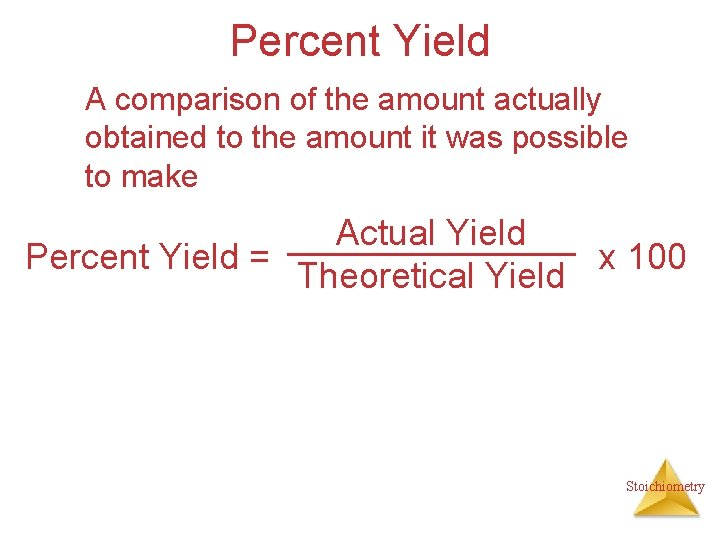Percent Yield A comparison of the amount actually obtained to the amount it was possible to make Actual Yield Percent Yield = x 100 Theoretical Yield Stoichiometry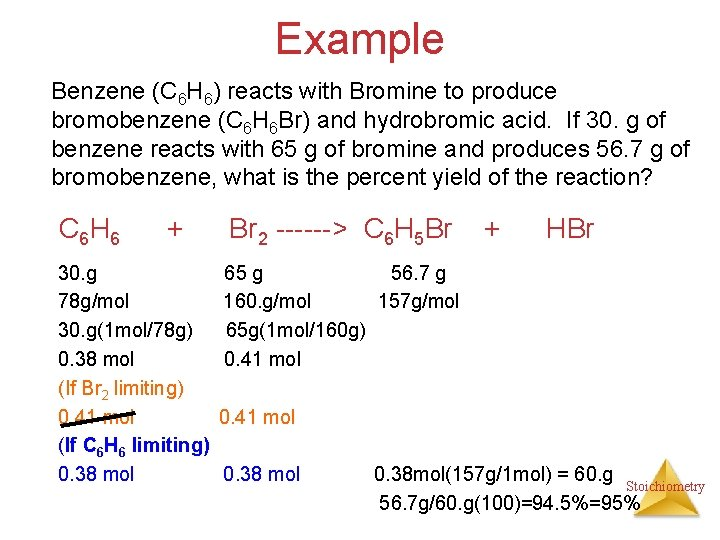Example Benzene (C 6 H 6) reacts with Bromine to produce bromobenzene (C 6 H 6 Br) and hydrobromic acid. If 30. g of benzene reacts with 65 g of bromine and produces 56. 7 g of bromobenzene, what is the percent yield of the reaction? C 6 H 6 + 30. g 78 g/mol 30. g(1 mol/78 g) 0. 38 mol (If Br 2 limiting) 0. 41 mol (If C 6 H 6 limiting) 0. 38 mol Br 2 ------> C 6 H 5 Br + HBr 65 g 56. 7 g 160. g/mol 157 g/mol 65 g(1 mol/160 g) 0. 41 mol 0. 38 mol(157 g/1 mol) = 60. g Stoichiometry 56. 7 g/60. g(100)=94. 5%=95%Percent Yield You prepared cookie dough to make 5 dozen cookies. The phone rings while a sheet of 12 cookies is baking. You talk too long and the cookies burn. You throw them out (or give them to your dog. ) The rest of the cookies are okay. How many cookies could you have made (theoretical yield)? How many cookies did you actually make to eat? (Actual yield) Stoichiometry 29Vocabulary Actual yield is the amount of product actually recovered from an experiment Theoretical (possible) yield is the maximum amount of product that could be produced from the reactant. Percent Yield is the actual yield compared to the maximum (theoretical yield) possible. Stoichiometry 30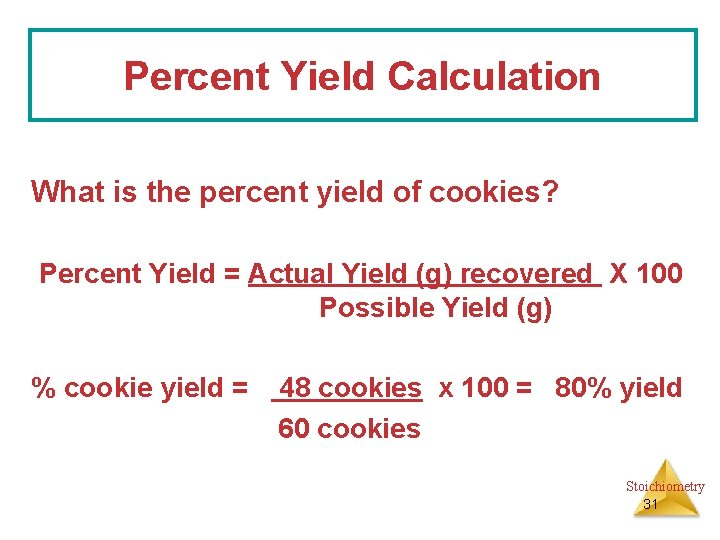Percent Yield Calculation What is the percent yield of cookies? Percent Yield = Actual Yield (g) recovered X 100 Possible Yield (g) % cookie yield = 48 cookies x 100 = 80% yield 60 cookies Stoichiometry 31Example, one more React 1. 5 g of NH 3 with 2. 75 g of O 2. How much NO and H 2 O is produced? What is left? 4 NH 3 + 5 O 2 ----> 1. 5 g 2. 75 g 17 g/mol 32 g/mol 1. 5 g(1 mol/17 g)= 2. 75 g(1 mol/32 g)=. 088 mol. 086 (If NH 3 limiting): . 088 mol. 088(5/4)=. 11 O 2 limiting: . 086(4/5)=. 086 mol. 069 mol(17 g/mol) 1. 2 g 2. 75 g 4 NO ? 30. g/mol + 6 H 2 O ? 18 g/mol . 086 mol(4/5)=. 086(6/5)=. 069 mol. 10 mol. 069 mol(30. g/mol). 10 mol(18 g/mol) 2. 1 g 1. 8 g StoichiometryStoichiometry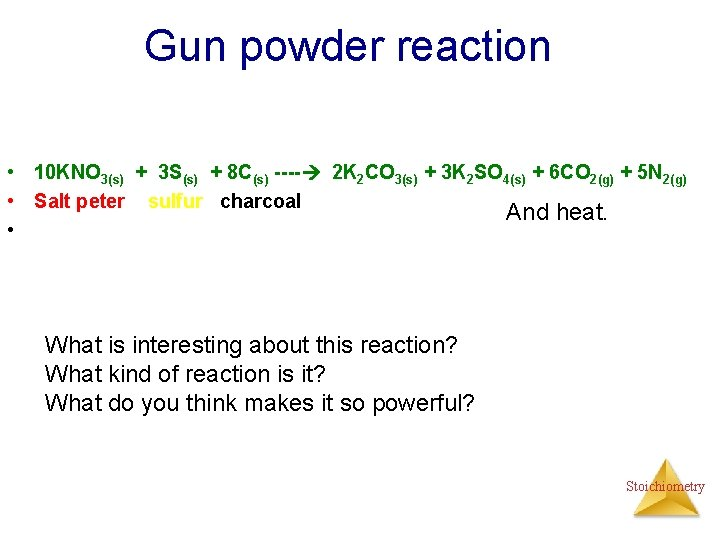Gun powder reaction • 10 KNO 3(s) + 3 S(s) + 8 C(s) ---- 2 K 2 CO 3(s) + 3 K 2 SO 4(s) + 6 CO 2(g) + 5 N 2(g) • Salt peter sulfur charcoal And heat. • What is interesting about this reaction? What kind of reaction is it? What do you think makes it so powerful? Stoichiometry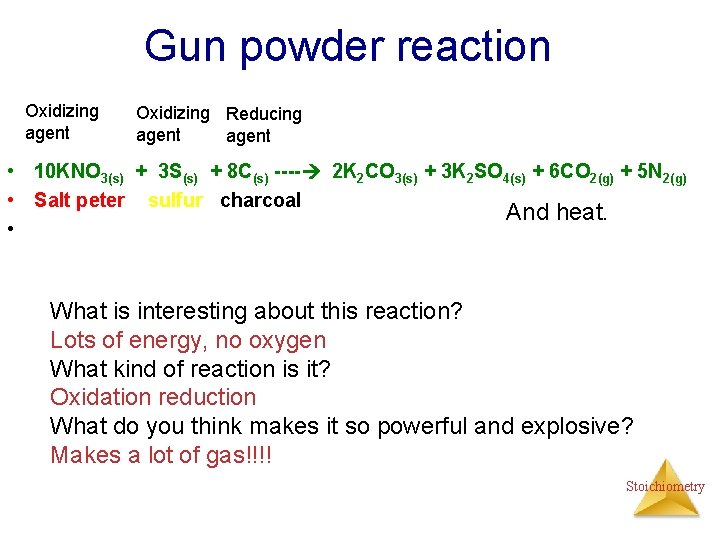Gun powder reaction Oxidizing agent Oxidizing Reducing agent • 10 KNO 3(s) + 3 S(s) + 8 C(s) ---- 2 K 2 CO 3(s) + 3 K 2 SO 4(s) + 6 CO 2(g) + 5 N 2(g) • Salt peter sulfur charcoal And heat. • What is interesting about this reaction? Lots of energy, no oxygen What kind of reaction is it? Oxidation reduction What do you think makes it so powerful and explosive? Makes a lot of gas!!!! Stoichiometry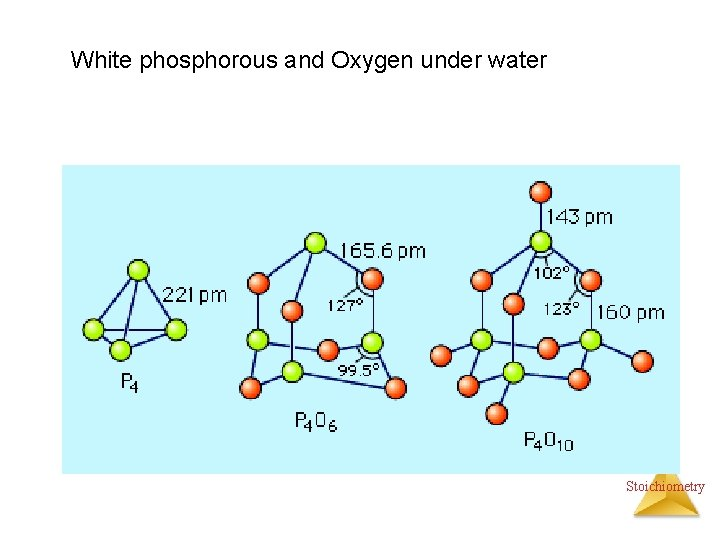White phosphorous and Oxygen under water Stoichiometry